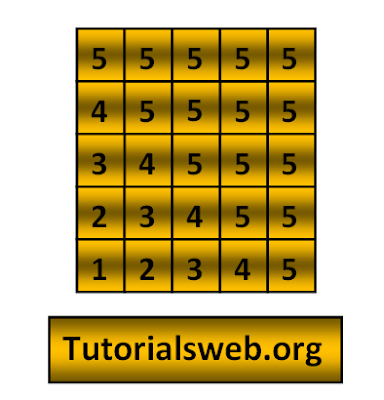## Write "C"  program to print below Number pattern 18:To understand this example, you should have knowledge of the following topics:
·      C programming operators
C Number pattern 18 program using For loop:
```
#include<stdio.h>
int main()
{
int i, j, k;
for(i=5;i>=1;i--)
{
k = i;
for(j=1;j<=5;j++)
{
if(k <= 5)
{
printf("%d",k);
}
else
{
printf("5");
}
k++;
}
printf("\n");
}
return 0;
}
```
Output:
`55555`
`45555`
`34555`
`23455`
`12345`
Explanation:
• First three integers "i", "j" and "k" are declared of type int.
• Then in the First for loop “i” value is initialized with a value 5.
• Now “i” value is checked with the condition i >= 1 that is (5 >= 1). So the condition is True.
• Now the loop enters into second for loop and checks the condition j <= 5. Where "j = 1". and the condition is True.
• Now Loop checks if condition "k <=5"  where "k = 5" if the condition is True prints the k value if the Condition is False prints "5". Print up to second for loop condition becomes False.
• when the value of "i"  becomes "0" the program terminates.